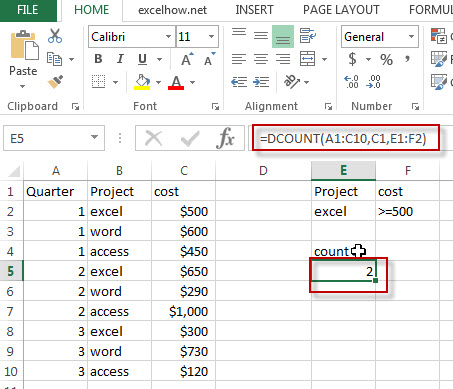# Excel Dcount Function

This post will guide you how to use Excel DCOUNT function with syntax and examples in Microsoft excel.

### Description

The Excel DCOUNT function returns the number of cells that containing numbers in a column of a list or a database that match the conditions that you specify.

The DCOUNT function is a build-in function in Microsoft Excel and it is categorized as a Database Function.

The DCOUNT function is available in Excel 2016, Excel 2013, Excel 2010, Excel 2007, Excel 2003, Excel XP, Excel 2000, Excel 2011 for Mac.

### Syntax

The syntax of the DCOUNT function is as below:

`= DCOUNT (database, field, criteria)`

Where the DCOUNT function arguments are:

• Database -This is a required argument.  The range of cells that containing the database.
• Field – This is a required argument.  The column to count the values.
• Criteria – The range of cells that contains the conditions that you specify.

### Excel DCOUNT Function Examples

The below examples will show you how to use Excel DCOUNT Function to count the cells that contain numbers in a column of a list or database that match a given criteria.

#1 =DCOUNT(A1:C10,C1,E1:F2)Note: The above excel formula will find excel project and counts how many of the cost fields in those records contains numbers.

### Related Functions

• Excel DcountA Function
The Excel DCOUNTA function returns the number of cells that containing nonblank values in a column of a list or a database that match the conditions that you specify.The syntax of the DCOUNTA function is as below:= DCOUNTA (database, field, criteria)…